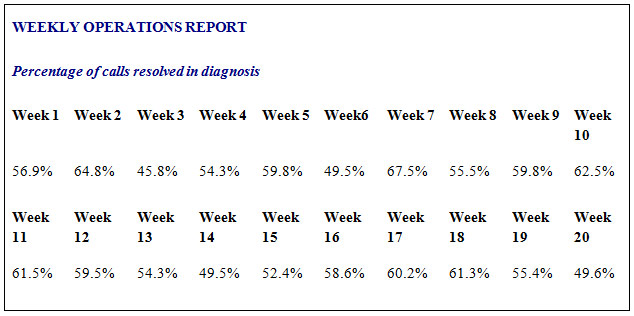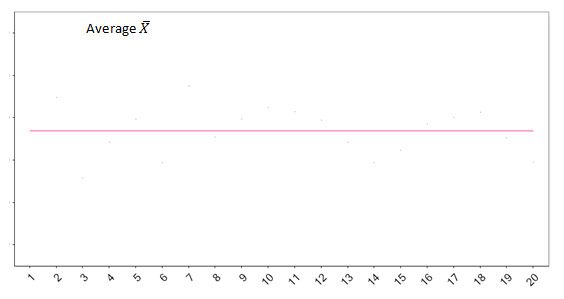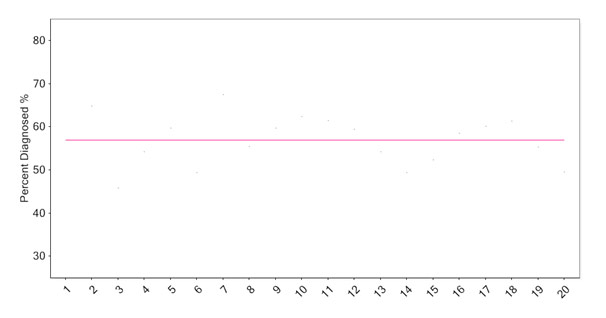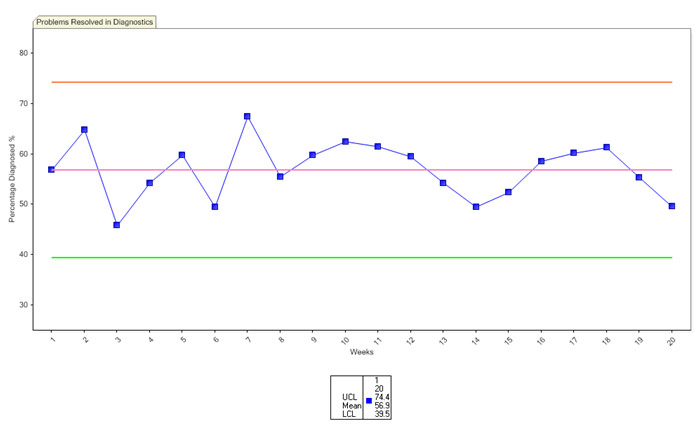-Construct an SPC chart using CapChart software
-Construct manually

This section will teach you how to make a chart manually.

The construction of a capability chart is very straightforward.  The type of measure you are plotting will decide what type of plot you choose to use. The essentials of the methodologies are, however, the same, as is the interpretation of the plot, only the calculation differs.  They are all based on an average of the numbers involved and the range (difference) between them.

The steps below will teach you how to create an X-bar Moving Range plot; don’t worry about the title, this is the chart you will need the most if you are studying a service organisation.

Below is a run of performance numbers.  In this case they are the monthly percentages of customer problems resolved in a front line unit.  Ideally, this organisation would like all customer problems to be resolved at this first point of contact without any having to be routed back into other parts of the organisation, which obviously makes things worse for the customer and incurs greater costs.Figure 3 – Extract from Weekly Operations Report

Step 1

Establish the average of this sequence of numbers. (To ensure a robust plot a sequence of a minimum of 20 data points is needed).  You know how to do this: divide the total by the number in the sequence. In this case the average is 56.9% ( X-bar = 56.9)
The average provides the centre line of the plot.  Draw a horizontal line to represent this.Figure 4 – Plot showing Average Line

Step 2

Determine an appropriate vertical scale for the plot.  In this case it will be a percentage scale. Make your scale on the vertical axis.Figure 5 – Plot showing Average Line and Vertical Scaling

Step 3

Determine the range of difference between the numbers in the sequence.  To do this, take the second number from the first, the third from the second and so on.  Doing this will sometimes result in negative numbers, i.e. where the number you are taking away is greater than the one you are taking it away from.

This does not matter.  Ignore the negative designation.  All you want to establish is the magnitude of the difference between them.

This will give you a second sequence of numbers, one less than the original sequence.  These numbers are referred to as the range (of difference) between the original numbers.

 % Solved in Diagnosis Range of difference 56.9 7.9 64.8 19.0 45.8 8.5 54.3 5.5 59.8 10.3 49.5 18.0 67.5 12.0 55.5 4.3 59.8 2.7 62.5 1.0 61.5 2.0 59.5 5.2 54.3 4.8 49.5 2.9 52.4 6.2 58.6 1.6 60.2 1.1 61.3 5.9 55.4 5.8 49.6

Figure 6 – The range of difference between the numbers in the original sequence

Step 4

Calculate the average of this second sequence of numbers (the ranges).  In this example this average is 6.56 (  R = 6.56).

Step 5

Multiply this average of the ranges (  R = 6.56) by 2.66.  (All you need to know at this time is that 2.66 is a constant which will give you three standard deviations* on either side of your original average which you have drawn as the centre line of the plot).

Step 6

Now add the result of your R x 2.66 calculation to the original average.  This will give you the distance of the upper control limit from the average on your plot: – draw it in.  And take it away from the original average which will give you the distance of the lower control limit from the original average: – draw it in.  See Figure 7.

A summary of the Steps
Average of % Diagnosed  = 1138.7/20 = 56.94%
Average of Range Difference = 124.7/19 = 6.56
Average of Range Difference x 2.66 = 6.56×2.66 = 17.46
Upper Control Limit = 56.94 + 17.46 = 74.39%
Lower Control Limit = 56.94 – 17.46 = 39.48%Figure 7 – Plot showing Average, Upper and Lower Control Limit

You now have a centre line which represents the average, and upper and lower control limits which indicate the degree of variation which is inherent in the current process performance.

* standard deviation = average deviation from the mean
Step 7

Enter the raw data of the original sequence onto the plot.  See figure 8 below.Figure 8 –  X- bar moving Range plot of problems resolved

You now have an SPC plot of the front line unit’s performance capability to resolve customer problems at this first point of contact.

Of course, the important thing is not so much the plot as the interpretation of the plot.